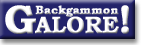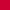RatingsForum Archive : Ratings

 Constructing a ratings system

 From: Matti Rinta-Nikkola Address: rintanikkola@phys.jyu.fi Date: 1 December 1998 Subject: The Backgammon rating system Forum: rec.games.backgammon Google: 3663EF61.9839D287@phys.jyu.fi

```I have done some studying about the Backgammon
rating formula used in many Backgammon servers.
I send the results of my thinking here... perhaps
someone might find use to them.

Best regards,
Matti Rinta-Nikkola

Backgammon rating formula
-------------------------
Many persons have noticed that the FIBS rating formula
(used also in many other backgammon servers) does not
work correctly for different match lengths. This conclusion
is done by studying match statistics collected from the
FIBS (ref 1,2,3,4,5). I will explain here how the rating
formula could be modified to be more accurate in different
match lengths. This problem has been studied also by many
others (ref 6,7).

1. FIBS rating formula
----------------------
The FIBS formula has been described elsewhere in more
detailed (ref 8). The main assumption of the rating formula
is that the rating distribution of the players will follow
the Gaussian distribution. In order to derive the formula
for the different match lengths it has been presumed that
the game winner get always one point (i.e. no gammons,
backgammons or doubling cube) (ref 8)! These assumptions
lead to the match winning probability formula:

1
P(D) =   ----------------------           ;
10**(-D*SQRT(Skill)/2000) + 1

where D is the elo difference of the players
P is the winning probability
Skill is the match length.

So what is wrong in the formula above? Formula itself is
correct but the second assumption that have been used to
derive it is wrong! Wrong assumption leads to the erroneous
Skill function. In Backgammon the Skill function is not
simply equal the match length.

2. Backgammon Skill function
----------------------------
Who will win most when you play Backgammon? If you mostly
lose your matches then its certainly luckier player who win
mostly :-). But if you win more you probably like to explain
the Backgammon playing skills you have. What those skills
might be? Obviously there is two skills: 1) checker play and
2) cube handling.

Lets us try to construct the Skill function for Backgammon
rating formula. As already noted above in FIBS formula the
Skill function is simply

Skill(N)= N ; where N is a match length

If we introduce the doubling cube to the game the average
points per game will increase and the checker play skill
become less important in a given match length. On the other
hand doubling cube brings the new skill to the play -cube
handling skill. Studying match equity table for players of
different checker play skills (ref 7) we see that
the the probability to win 1 point match is equal to that
of two point match as well as the probability of winning
3 and 4 point matches are equal. Because of that fact it is
easier to construct the Skill function separately for even
and odd point matches. Here I will consider only odd point
matches i.e. N=1,3,5,7... . For odd point matches the Skill
function can be written as

N-1
Skill(N)= 1 + (cp + ch)( --- ) ;   N=1,3,5,7...  (1)
2

where cp defines the "extra" checker play skill in a matches
(N>1)
ch defines the cube handling skill

o Note 1: If the cube handling skill ch=0 the Skill
function gives the expectation value of the minimum
number of games needed to win the match i.e.

Skill(N) = N/ppg(N) ;   ch=0                   (2)

where ppg(N) is the average points per game. In a longer
matches ppg(N) is near the value of the ppg in a money game.
The value of cp can be calculated using equations (1) and
(2).

o Note 2: Cube handling and checker play skill parameters
(ch, cp) are expressed in units of the one point match
checker play skill.

o Note 3: Total checker play and cube handling skills in
a N point match are 1+cp*(N-1)/2 and ch*(N-1)/2.

3. Defining the values for parameters cp and ch
-----------------------------------------------

- Checker play skill

As noted before the cp can be calculated if the cube
handling skill is equal zero. From equations (1) and (2)
we get

cp=2*(N/ppg(N) - 1)/(N-1) = 2/ppg  ; assuming N>>1  (3)

The cp value for shorter matches (N=3,5,7...) is a bit
smaller than the value obtained from the equation (3).
Better estimation for cp is got if we use smaller N, for
example N=21. If the ppg=1 as assumed in the derivation
of the FIBS rating formula we will get cp=2.

More realistic value can be obtained if we assume continues
Backgammon and efficient doubling (assumptions used to
derive match equity table). In that case ppg=3.3 and we can
calculate cp from the equation (3) (cube handling skill is
zero because cube handling errors are not made). We obtain
cp=0.61. More accurate value for cp can be obtained if the
Skill function is fitted to the match equity data, see
table 1. I have used match equity table calculated by Tom Keith
(ref 7).

We can make another estimation for cp if we assume that the
JellyFish is playing perfect Backgammon (at level 5:)).  For
JellyFish ppg=2.3 (ref 9) which gives cp = 0.87.

The rolls method introduced by Tom Keith (ref 7) gives c=0.84,
see Table 1. The rolls method does not give any information
the cube handling errors are averaged out from the used data

We can calculate the checker play skill from equation (3) also
in a case when the match is played without doubling cube.
Assuming 25% gammon rate we can calculate cube less
ppg = 0.75+2*0.25 = 1.25 which gives cp=1.6

Table 1. Skill function for different methods (ch=0). In
parenthesis is the value calculated from the
equation (1) using the fitted cp value.

FIBS       MatEq         Rolls      JellyFish
Match length 1     1  (1)   1.00  (1.00)   1.00  (1.00)
Match length 3     3  (3)   1.54  (1.54)   1.77  (1.84)
Match length 5     5  (5)   2.07  (2.08)   2.66  (2.68)
Match length 7     7  (7)   2.62  (2.62)   3.57  (3.52)
Match length 9     9  (9)   3.13  (3.16)   4.67  (4.36)
Match length 11   11 (11)   3.69  (3.70)   5.48  (5.20)
fitted cp            2         0.54           0.84
calculated cp        2         0.61            -           0.87

- Cube handling skill

The value of the ch parameter has to be calculated from the
experimental data. There is no way to determine it theoretically
because its value depends on the cube handling and checker play
errors players do. ch depends on the checker play errors too
because it has to be expressed in the same units as cp.

However we already know that the FIBS value for cp+ch=2 is too
high and we have also very good estimation for cp=0.85. So we
must have ch < 1.15.

One limit can be still found if there is someone who is able to
answer to the following question: Lets assume that the top rated
player plays an 11 point match against an averaged rated player.
Who get the advantage in the match if they decide to play without
doubling cube?
As calculated above we know that cp=1.6 in a match played without
doubling cube. If the answer to the above question is "Top rated
player", which I think is the correct answer, we can write

P        >  P
nocube      cube

<=>
Skill        > Skill
nocube         cube

=>  ch < 0.75

4. Backgammon rating system
---------------------------
Finally I will suggest how the rating system should be implemented
in the Backgammon server.

1) I think that the rating system should be simplified so that the
rating is calculated only for odd point matches (N=1,3,5,7...)
2) Pick up a value for "cp+ch". It should be in the range from 0.8
to 2.0. In a new server I would probably start with a value
cp+ch=1.2. In a old server like FIBS I think you need to ask from
the players what they think about. Perhaps they don't want to
change the rating system at all :-).
3) Design the system which can indicate easily if the parameter cp+ch
has a wrong value.
4) Be conservative when changing the value cp+ch and don't change it
too often.

References
----------
1) FIBS--Rating Formula:  Different length matches by Jim Williams
http://www.bkgm.com/rgb/rgb.cgi?view+603
2) FIBS--Rating Formula:  Emperical analysis by Carry Wong
http://www.bkgm.com/rgb/rgb.cgi?view+601
3) FIBS--Rating Formula  One-point matches by David Montgomery
http://www.bkgm.com/rgb/rgb.cgi?view+44
4) FIBS--Rating Formula  Opponent's strength by William Hill
http://www.bkgm.com/rgb/rgb.cgi?view+524
5) Match Archives  Big Brother--Statistics by Peter Fankhauser
http://www.bkgm.com/rgb/rgb.cgi?view+139
6) FIBS--Rating Formula  Possible adjustments by Christopher D. Yep
http://www.bkgm.com/rgb/rgb.cgi?view+597
7) FIBS--Rating Formula  Different length matches by Tom Keith
http://www.bkgm.com/rgb/rgb.cgi?view+523
8) ELO ranking
http://www.netgammon.com/us/facts/elo2.htm
9) Miscellaneous  Distribution of points per game by Stig Eide
http://www.bkgm.com/rgb/rgb.cgi?view+513
```

### Ratings

Constructing a ratings system  (Matti Rinta-Nikkola, Dec 1998)
Converting to points-per-game  (David Montgomery, Aug 1998)Cube error rates  (Joe Russell+, July 2009)Different length matches  (Jim Williams+, Oct 1998)
Different length matches  (Tom Keith, May 1998)ELO system  (seeker, Nov 1995)
Effect of droppers on ratings  (Gary Wong+, Feb 1998)
Emperical analysis  (Gary Wong, Oct 1998)
Error rates  (David Levy, July 2009)
Experience required for accurate rating  (Jon Brown+, Nov 2002)
FIBS rating distribution  (Gary Wong, Nov 2000)
FIBS rating formula  (Patti Beadles, Dec 2003)
FIBS vs. GamesGrid ratings  (Raccoon+, Mar 2006)Fastest way to improve your rating  (Backgammon Man+, May 2004)
Field size and ratings spread  (Daniel Murphy+, June 2000)Improving the rating system  (Matti Rinta-Nikkola, Nov 2000)KG rating list  (Daniel Murphy, Feb 2006)KG rating list  (Tapio Palmroth, Oct 2002)
MSN Zone ratings flaw  (Hank Youngerman, May 2004)
No limit to ratings  (David desJardins+, Dec 1998)
On different sites  (Bob Newell+, Apr 2004)
Opponent's strength  (William Hill+, Apr 1998)
Possible adjustments  (Christopher Yep+, Oct 1998)
Rating versus error rate  (Douglas Zare, July 2006)Ratings and rankings  (Chuck Bower, Dec 1997)Ratings and rankings  (Jim Wallace, Nov 1997)
Ratings on Gamesgrid  (Gregg Cattanach, Dec 2001)
Ratings variation  (Kevin Bastian+, Feb 1999)
Ratings variation  (FLMaster39+, Aug 1997)
Ratings variation  (Ed Rybak+, Sept 1994)
Strange behavior with large rating difference  (Ron Karr, May 1996)
Table of ratings changes  (Patti Beadles, Aug 1994)
Table of win rates  (William C. Bitting, Aug 1995)
Unbounded rating theorem  (David desJardins+, Dec 1998)
What are rating points?  (Lou Poppler, Apr 1995)
Why high ratings for one-point matches?  (David Montgomery, Sept 1995)From GammOnLineLong messageRecommended readingRecent addition

 Book Suggestions Books Cheating Chouettes Computer Dice Cube Handling Cube Handling in Races Equipment Etiquette Extreme Gammon Fun and frustration GNU Backgammon History Jellyfish Learning Luck versus Skill Magazines & E-zines Match Archives Match Equities Match Play Match Play at 2-away/2-away Miscellaneous Opening Rolls Pip Counting Play Sites Probability and Statistics Programming Propositions Puzzles Ratings Rollouts Rules Rulings Snowie Software Source Code Strategy--Backgames Strategy--Bearing Off Strategy--Checker play Terminology Theory Tournaments Uncategorized Variations